# Calculating the DLOG Area Size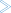To calculate a DSF logging area size (DLOGSIZE), use the following procedure as a guide:

1. First, determine

• the estimated number of Associator/Data Storage write I/O operations that will occur between consecutive delta save operations (WIOS);

• the Associator data set block size (ASSOBSIZ) for the Associator device from the tables in appendix A of the Adabas Operations documentation, and

• the security factor (DLOGFAC), a multiplier to ensure that no DLOG overrun occurs.

2. Calculate the approximate number of logged RABNs per DLOG block (LOGRABNS):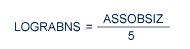3. Calculate the needed number of DLOG detail blocks (DLOGBLKS):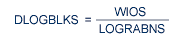4. Calculate the DLOG block count (DLOGRAW), including the security factor:

```
DLOGRAW = DLOGBLKS * DLOGFAC

```

The value DLOGSIZE is the calculated size of the DLOG area, in blocks.

#### Example:

The DLOG size is calculated using an average number of I/O operations between Delta Save operations of 1,000,000 based on a 3390 device type:

The approximate number of logged RABNs per block is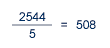The required detail blocks are calculated as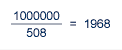The total DLOG block count including the security factor (in this case, 3) is calculated as

```
1968 * 3 = 5904

```

or 5904 blocks of DLOG area.

This value is entered in the "DLOG Area Size" field.